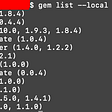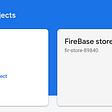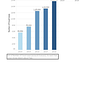# AutoML Library for Regression : AutoKeras

What is AutoKeras?

It is an open-source AutoML system based on Keras.

What’s AutoML ? Well, it will make your life easy by finding out the best-performing model for a given dataset.

We will look at how it can done.

Install AutoKeras :

`!pip install autokeras`

Import Necessary Modules :

`import numpy as npimport pandas as pdimport plotly.express as pxfrom autokeras import StructuredDataRegressorfrom sklearn.model_selection import train_test_splitfrom IPython.display import HTML`

Let’s take a simple sales data ,

`df = pd.read_csv("Data.csv")df.head()`

Split Data :

`target_col = "sales"X = df.loc[:, df.columns != target_col]y = df.loc[:, target_col]# Train test split X_train, X_test, y_train, y_test = train_test_split(X, y,                                                     test_size=0.20,                                                     random_state=2021)`

Now let’s train our model, Autokeras will find out the best possible model, this is achieved by StructuredDataRegressor class defined by AutoKeras library.

`# max trials tries 15 different models, MAE is the loss functionsearch = StructuredDataRegressor(max_trials=15, loss='mean_absolute_error')# Fitting our training data to the model class search.fit(x=X_train, y=y_train)# You can also add Keras Callbacks while fitting to model classtensorboard_callback = tf.keras.callbacks.TensorBoard(log_dir='./logs', histogram_freq=0,write_graph=True, write_images=False)search.fit(x = X_train, y = y_train, epochs = 50,callbacks = [tensorboard_callback], validation_data=(X_test,y_test))`

This can take a few minutes depending on the system..

Evaluate the model on train and test data to see how it performs :

`train_mae, _ = search.evaluate(X_train, y_train, verbose=0)print('Mean Absolute Error is {}'.format(train_mae))test_mae, _ = search.evaluate(X_test, y_test, verbose=0)print('Mean Absolute Error is {}'.format(test_mae))`

Save the model :

`model = search.export_model()# To view the model summarymodel.summary()try:model.save("model_autokeras", save_format="tf")except Exception:model.save("model_autokeras.h5")`

Load the saved model for prediction :

`from tensorflow.keras.models import load_modelloaded_model = load_model("model_autokeras", custom_objects=autokeras.CUSTOM_OBJECTS)predicted_y = loaded_model.predict(tf.expand_dims(X_test, -1))`

Hope you learned something new today, Happy Learning!

--

--

--

## More from Amal

Regular Post | Data Scientist, India

Love podcasts or audiobooks? Learn on the go with our new app.

## How to Deploy a Dockerised Application on AWS ECS With Terraform## Linux File Permissions## How to use multiple cocoapod version on same Mac machine.## • My experiences with debugging skills, how they contribute to my success so far..## “Edge Computing ” Science-Research, January 2022, Week 1 — summary from Arxiv, Astrophysics Data…## iOS Firebase Part 1 (Cloud Firestore)## Apache Iceberg : A Primer## Amal

Regular Post | Data Scientist, India

## CREDIT CARD FRAUD DETECTION: A CONTEMPORARY PERSPECTIVE IN THE FIELD OF DATA SCIENCE## Correlation Analysis## Empowering Comet with GitLab